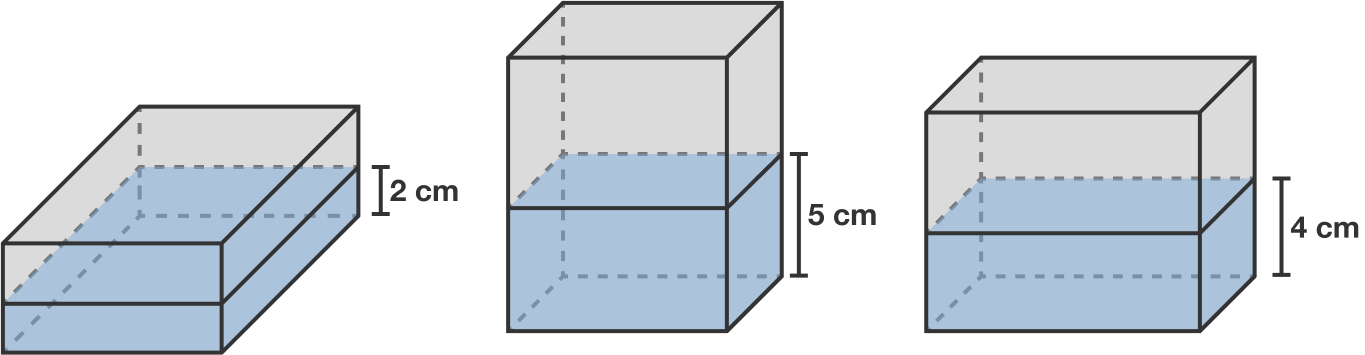# Glass Box

A cuboid water tank is partially filled with $\SI{160}{\centi\meter}^3$ of water. Depending on which face it rests on, the height of water in the tank is $\SI{2}{\centi\meter}, \SI{5}{\centi\meter},$ or $\SI{4}{\centi\meter}.$

What is the volume of this tank $\big($in $\si{\centi\meter\cubed}\big)?$

Note: The diagram is not drawn to scale.×